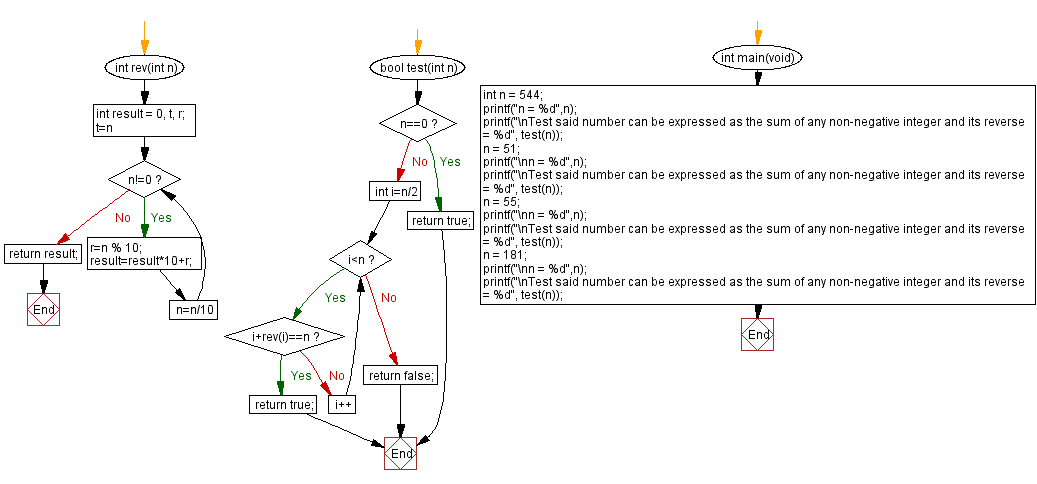﻿ C - Number sums and their reverses

# C Programming: Number sums and their reverses

## C Programming Mathematics: Exercise-32 with Solution

Write a C programming to check whether a given integer can be expressed as the sum of any non-negative integer and its reverse. Return true otherwise false.

Example:
Input: = 554
Output: true
Explanation: 371 + 173 = 554
Input: = 51
Output: false
Explanation: It cannot be expressed as per said condition.
Input: = 55
Output: true
Explanation: 41 + 14 = 55
Input: = 181
Output: true
Explanation: 140 + 041 = 181

Test Data:
(554) -> 1
(51) -> 0
(55) -> 1
(181) -> 1

Sample Solution:

C Code:

``````#include <stdio.h>
#include <stdlib.h>
#include <string.h>

int rev(int n){
int result = 0, t, r;
for(t=n;n!=0;n=n/10){
r=n % 10;
result=result*10+r;
}
return result;
}

bool test(int n){
if(n==0) return true;
for(int i=n/2;i<n;i++){
if(i+rev(i)==n){
return true;
}
}
return false;
}

int main(void) {
int n = 544;
printf("n = %d",n);
printf("\nTest said number can be expressed as the sum of any non-negative integer and its reverse = %d", test(n));
n = 51;
printf("\nn = %d",n);
printf("\nTest said number can be expressed as the sum of any non-negative integer and its reverse = %d", test(n));
n = 55;
printf("\nn = %d",n);
printf("\nTest said number can be expressed as the sum of any non-negative integer and its reverse = %d", test(n));
n = 181;
printf("\nn = %d",n);
printf("\nTest said number can be expressed as the sum of any non-negative integer and its reverse = %d", test(n));
}
```
```

Sample Output:

```n = 544
Test said number can be expressed as the sum of any non-negative integer and its reverse = 1
n = 51
Test said number can be expressed as the sum of any non-negative integer and its reverse = 0
n = 55
Test said number can be expressed as the sum of any non-negative integer and its reverse = 1
n = 181
Test said number can be expressed as the sum of any non-negative integer and its reverse = 1

```

Flowchart:C Programming Code Editor:

Improve this sample solution and post your code through Disqus.

What is the difficulty level of this exercise?

Test your Programming skills with w3resource's quiz.

﻿